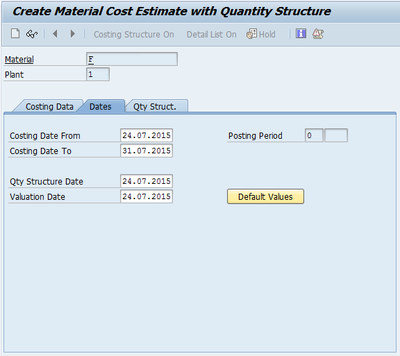Search
•admin

# SAP Costing Variant – Part I (Overview)

## SAP Costing Variant

We have already gone through the series of posts explaining about  the Costing Sheet previously. Hopefully, it helped you understand product costing a little more. SAP as we know is an ocean and now let me take your some time to discuss more  on product costing part which is the essence of SAP.

In this post we will discuss about Costing Variant which forms a very important part of SAP’s product costing.

A Costing Variant is the main element of SAP’s configuration which plays vital role in Cost Estimates. We will see how.

## Standard Cost Estimate

Before we go ahead, we will first see how a Screen of Creating a Material Cost Estimate looks like (refer Image 1.01 and 1.02)Standard Cost Estimate (Image 1.01)Standard Cost Estimate (Image 1.02)

We all are very familiar with the above screens. The above screens are part of our periodic process of creating and saving the Standard Cost Estimates for Materials. Here in the Image 1.01 we can see that we have entered the Costing Variant. After entering the Costing Variant when we go to the next screen (Image 1.02), we find some dates already provided by the system. This and many other parameters are controlled by Costing Variant. A Costing Variant contains all the control parameters required for costing.

### Costing Variants

SAP provides standard Costing Variant, given below is a brief explanation about them:

### PPC1: Standard Cost Estimate

A standard cost estimate calculates the standard costs for a semifinished or finished product. Costing type 01 specifies that the results of this cost estimate are written to the material master as the standard price, and thus can be used to valuate inventories. Costing variant PPC1 specifies valuation variant 001, quantity structure determination ID PC01, and date control PC01. No transfer control takes place.

### PPC2: Modified Standard Cost Estimate

You create a modified standard cost estimate when the data for costing has changed within a planning period. Costing type 12 specifies that the results of this cost estimate are used only for informational purposes. Costing variant PPC2 specifies valuation variant 009, quantity structure determination PC01, and date control PC05.

### PPC3: Current Cost Estimate

You create a current cost estimate when you want to make a decision based on the current price of the material. Costing type 13 specifies that the results of this cost estimate are used only for informational purposes. As an alternative, you can set the indicator Prices other than standard price in the costing type so that the results of this cost estimate can be written to the material master as a planned price. Costing variant PPC3 specifies valuation variant 009, quantity structure determination PC01, and date control PC04.

### PREM: Preliminary Costing of Cost Collectors

The preliminary cost estimate for the product cost collector is used to calculate preliminary costs on the basis of the quantity structure of a production process. Costing type 19 means this cost estimate is only relevant for product cost collectors. Costing variant PREM specifies valuation variant 001, transfer control PC02, and date control PC01. Quantity structure determination is not relevant, as quantity structure is determined through the production process.

Once we have had a little overview about Costing Variant, we will start looking deeper into the Costing variant. For this, lets see how costing variant PPC1 looks and works like (refer Image 1.03).Costing Variant (Image 1.03)

In the above image we can see that the Costing Variant is not a single configuration, instead a combination of various tabs representing different aspects of product costing consisting of various components. So we can see that a costing variant is a combination of various components put together for product cost estimate.

In the next few posts in this series we will try to analyse, understand and discuss upon the various components that make a costing variant.

Costing Variant